# QlikView Creating Analytics

Discussion Board for collaboration related to Creating Analytics for QlikView.

HighlightedNot applicable

## Control start

Hi All,

I have a SPC chart (control chart) with categories with values as a line chart.

If my Category1, Category2, Category 3, Category 4 has same values like 200,201,202,203, means continuously 4 values are continuously  going up in that case I want to highlight these values in red (as circles).

Just like the same if the continuous values going down as per above example 197,196,195,194 in that case also it should highlight in red circles also I want to check if 3 or more continuous values are going up or going down it should highlight in circles.

Need help, urgent.

@Michael Tarralo.

Tags (1)
1 Solution

Accepted SolutionsMVP

## Re: Control start

Hi,

some other background colour function restricted to a second expression that only draws symbols could be:

```If(Above(Exp2,3)<Above(Exp2,2) and Above(Exp2,2)<Above(Exp2,1) and Above(Exp2,1)<Above(Exp2,0) or
Above(Exp2,2)<Above(Exp2,1) and Above(Exp2,1)<Above(Exp2,0) and Above(Exp2,0)<Below(Exp2,1) or
Above(Exp2,1)<Above(Exp2,0) and Above(Exp2,0)<Below(Exp2,1) and Below(Exp2,1)<Below(Exp2,2) or
Above(Exp2,0)<Below(Exp2,1) and Below(Exp2,1)<Below(Exp2,2) and Below(Exp2,2)<Below(Exp2,3)
,LightRed(255)
,Color(1)
)

```working for any number greater or equal 4 of consecutive increasing values: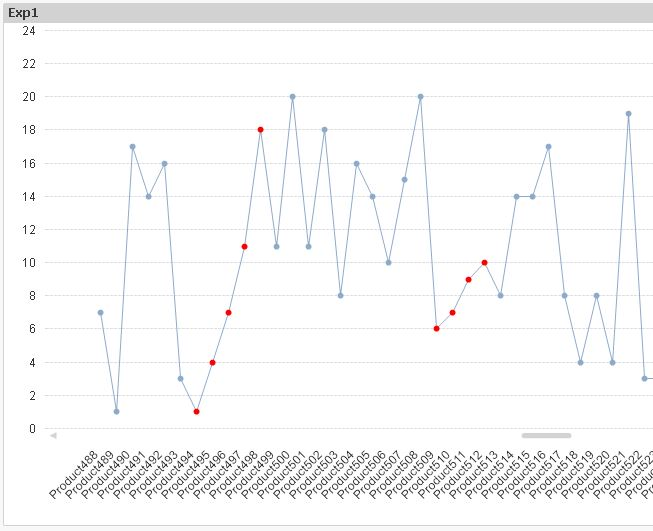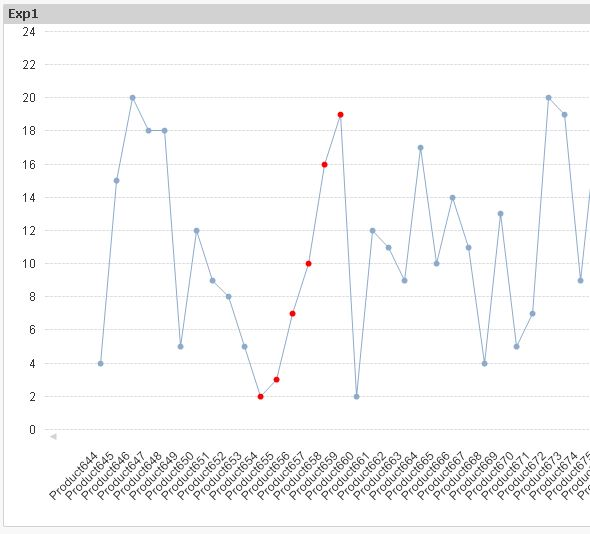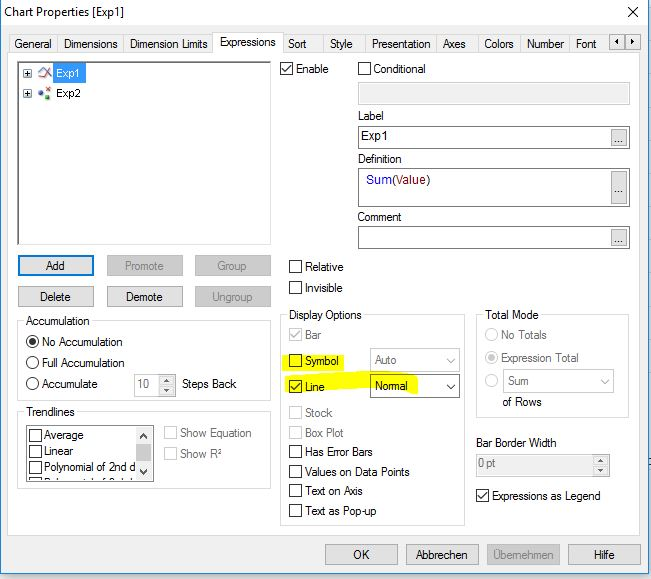test data:

```table1:
Ceil(Rand()*20) as Value
AutoGenerate 1000;
```

hope this helps

regards

Marco

8 RepliesMVP

## Re: Control start

Please post an example document with your data and - if possible - what you have accomplished already.

Also keep in mind that "urgent" has no real meaning in this community...PeterNot applicable

## Re: Control start

Hi Peter,

Thanks for the quick response.

In below I have Product column with different products with their values.

In below Line chart I have continuously values are increasing in this case I want to highlight those 4 values in red.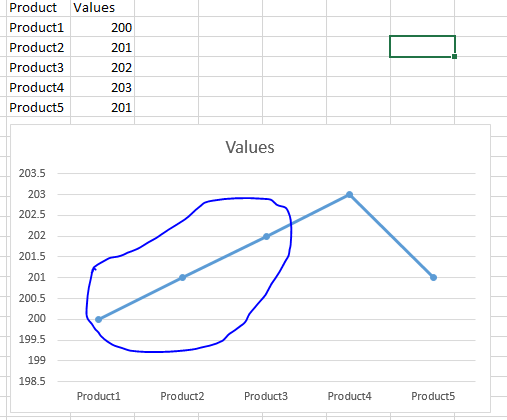Partner

## Re: Control start

Maybe something like this in your chart expressions background color attribute:

if( Below([Value]) > [Value], LightRed(),

if( Above([Value]) < [Value], LightRed() )

)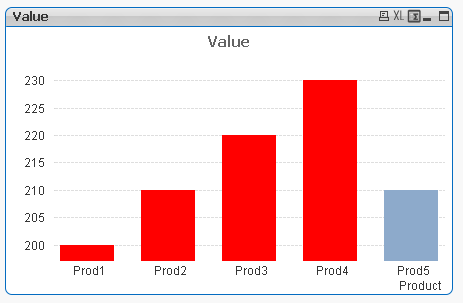MVP

## Re: Control start

Hi,

some other background colour function restricted to a second expression that only draws symbols could be:

```If(Above(Exp2,3)<Above(Exp2,2) and Above(Exp2,2)<Above(Exp2,1) and Above(Exp2,1)<Above(Exp2,0) or
Above(Exp2,2)<Above(Exp2,1) and Above(Exp2,1)<Above(Exp2,0) and Above(Exp2,0)<Below(Exp2,1) or
Above(Exp2,1)<Above(Exp2,0) and Above(Exp2,0)<Below(Exp2,1) and Below(Exp2,1)<Below(Exp2,2) or
Above(Exp2,0)<Below(Exp2,1) and Below(Exp2,1)<Below(Exp2,2) and Below(Exp2,2)<Below(Exp2,3)
,LightRed(255)
,Color(1)
)

```working for any number greater or equal 4 of consecutive increasing values:test data:

```table1:
Ceil(Rand()*20) as Value
AutoGenerate 1000;
```

hope this helps

regards

MarcoMVP

## Re: Control start

and some script solution with slider to select the minimum number of consecutive increasing values to mark red: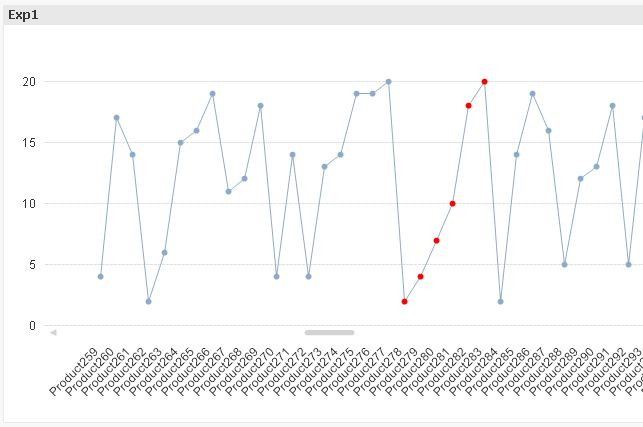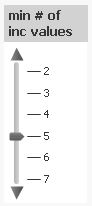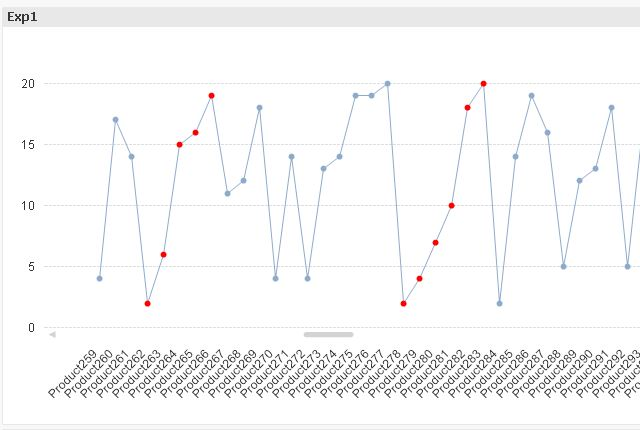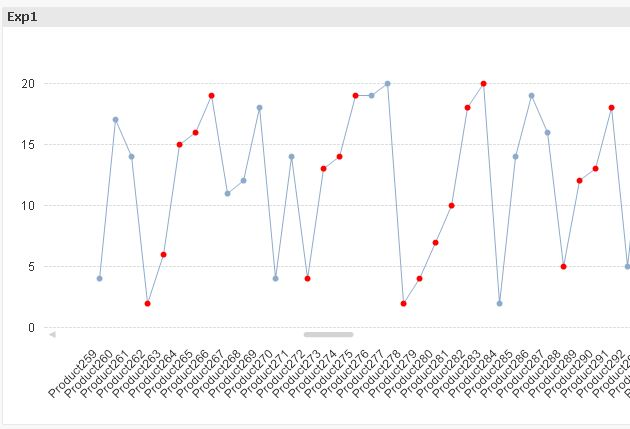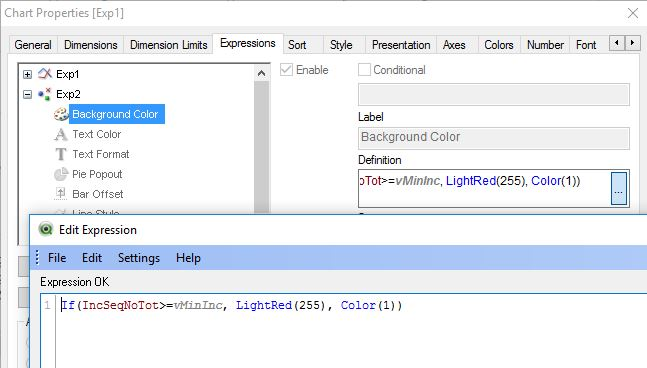```table1:
If(Value>Previous(Value),Alt(Peek(IncSeqNo)+1,1),0) as IncSeqNo;
Ceil(Rand()*20) as Value
AutoGenerate 1000;

Left Join (table1)
If(IncSeqNoInv+IncSeqNo, IncSeqNoInv+IncSeqNo+1) as IncSeqNoTot;
If(Value<Previous(Value),Alt(Peek(IncSeqNoInv)+1,1),0) as IncSeqNoInv
Resident table1
Order By Product desc;
```

hope this helps

regards

MarcoNot applicable

## Re: Control start

Hi Oscar,

I am almost achieved with your answer, but here I want to show if continuously minimum 7 values going up or down.

Thanks for the help. I really appreciate it.Not applicable

## Re: Control start

Hi Marco,

Really thanks for the response and I really appreciate it, I am almost to my requirement what I am looking for, but need some help so that I can achieve fully successfully..

If(Above(Exp2,3)<Above(Exp2,2) and Above(Exp2,2)<Above(Exp2,1) and Above(Exp2,1)<Above(Exp2,0) or

Above(Exp2,2)<Above(Exp2,1) and Above(Exp2,1)<Above(Exp2,0) and Above(Exp2,0)<Below(Exp2,1) or

Above(Exp2,1)<Above(Exp2,0) and Above(Exp2,0)<Below(Exp2,1) and Below(Exp2,1)<Below(Exp2,2) or

Above(Exp2,0)<Below(Exp2,1) and Below(Exp2,1)<Below(Exp2,2) and Below(Exp2,2)<Below(Exp2,3)    ,LightRed(255)   ,Color(1)  )

Here I want to assign a variable to constant value like in the above condition you have mentioned 3,2,1 so on, so if I give 7 in variable if the series of points (min 7) going up or down it should highlight.MVP

## Re: Control start

Hi,

instead of the script approach some front end solution could be to use a dynamic variable like this: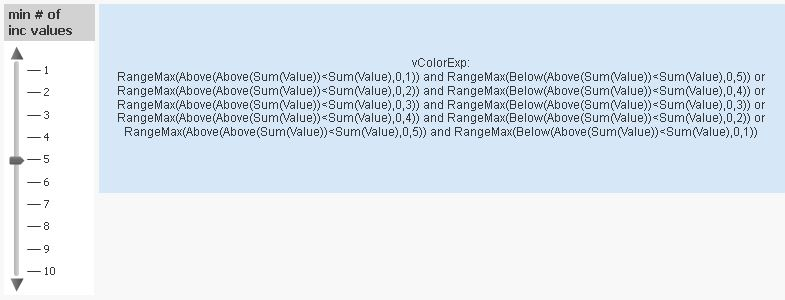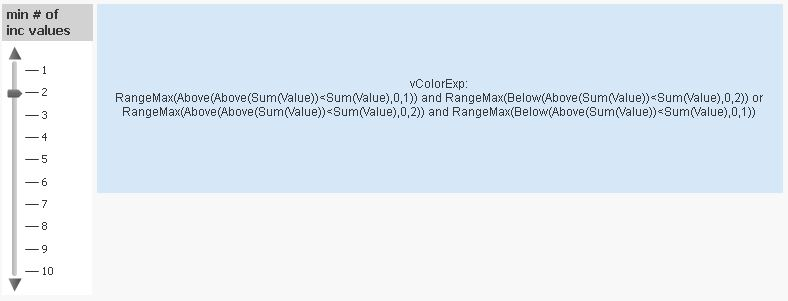Colour variable definition and sample data:

```LET vColorExp = '=Concat(''RangeMax(Above(Above(Sum(Value))<Sum(Value),0,''&ValueLoop(1,vMinInc)&'')) and RangeMax(Below(Above(Sum(Value))<Sum(Value),0,''&(vMinInc+1-ValueLoop(1,vMinInc))&''))'',   '' or '')';

table1: